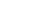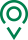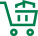• EN
• 圣康首页
• {if condition="\$categorys[\$vo]['is_menu'] == 1"}
• 关于圣康
• {if condition="\$categorys[\$vo]['is_menu'] == 1"} {if condition="\$categorys[\$vo]['is_menu'] == 1"}
• 产品展示
• {if condition="\$categorys[\$vo]['is_menu'] == 1"} {if condition="\$categorys[\$vo]['is_menu'] == 1"}
• 荣誉资质
• {if condition="\$categorys[\$vo]['is_menu'] == 1"} {if condition="\$categorys[\$vo]['is_menu'] == 1"}
• 新闻资讯
• {if condition="\$categorys[\$vo]['is_menu'] == 1"} {if condition="\$categorys[\$vo]['is_menu'] == 1"}
• 联系我们
• {if condition="\$categorys[\$vo]['is_menu'] == 1"} {if condition="\$categorys[\$vo]['is_menu'] == 1"}
• 在线留言
• {if condition="\$categorys[\$vo]['is_menu'] == 1"} {if condition="\$categorys[\$vo]['is_menu'] == 1"}
• 质量管控
• {if condition="\$categorys[\$vo]['is_menu'] == 1"} {if condition="\$categorys[\$vo]['is_menu'] == 1"}
• 源头种植
• {if condition="\$categorys[\$vo]['is_menu'] == 1"} {if condition="\$categorys[\$vo]['is_menu'] == 1"}
• 过程控制
• {if condition="\$categorys[\$vo]['is_menu'] == 1"}

# “一带一路”高校食品教育科技联盟在无锡成立

“中国有句古训，叫‘民以食为天’，也就是说，没有什么比食品问题更加重要。这是国际社会共性的挑战，也是我们共同的发展机遇。江南大学愿与大家一起，把握时代发展机遇，深化教育科技合作，实现共同繁荣进步。”论坛上，江南大学党委书记朱庆葆如是表示。

“我们希望通过联盟，广泛推进协同育人体系建设，建立优势学科交流机制，推进联合科研攻关和成果转化、加强师资队伍交流合作，共建‘互知互信、互帮互助、互学互鉴’的食品教育科技共同体，共同引领和促进‘一带一路’沿线各国，食品教育、食品科技与食品产业的全面发展。”朱庆葆称，“一花独放不是春，百花齐放春满园”，该校发起“一带一路”食品高校教育科技联盟倡议，得到了众多国际同仁的积极响应与共鸣。

### 我们的宗旨

•全球精选

一站式购物方案

•多仓直发

特有次日达配送服务

•源头直采

精选正宗好食材

•天天底价

新鲜到家购不停